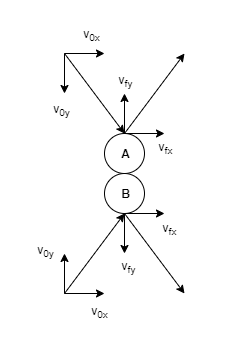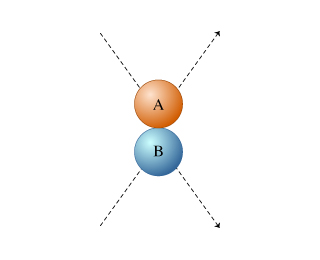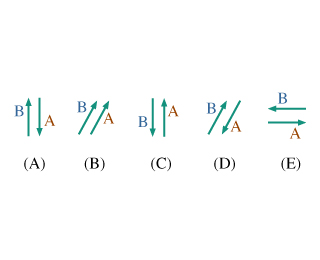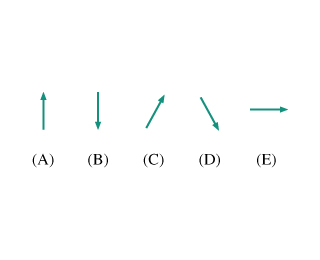# Problem: Balls A and B roll across a table, then collide and bounce off each other. The paths of the two balls are pictured (viewed from above) in the diagram. (Figure 1) a) Which set of arrows best represents the change in momentum for balls A and B?b) Which of the following arrows indicates the direction of the impulse applied to ball A by ball B?

###### FREE Expert Solution

a)

Change in momentum:

$\overline{){\mathbf{∆}}{\mathbf{p}}{\mathbf{=}}{\mathbf{m}}{\mathbf{∆}}{\mathbf{v}}}$

We'll break the velocity into x and y components.88% (416 ratings)###### Problem Details

Balls A and B roll across a table, then collide and bounce off each other. The paths of the two balls are pictured (viewed from above) in the diagram. (Figure 1)a) Which set of arrows best represents the change in momentum for balls A and B?b) Which of the following arrows indicates the direction of the impulse applied to ball A by ball B?